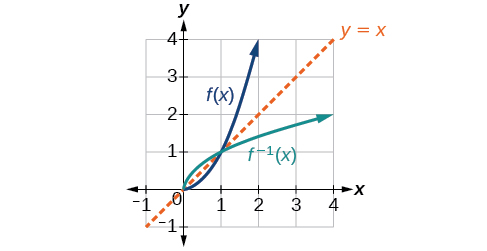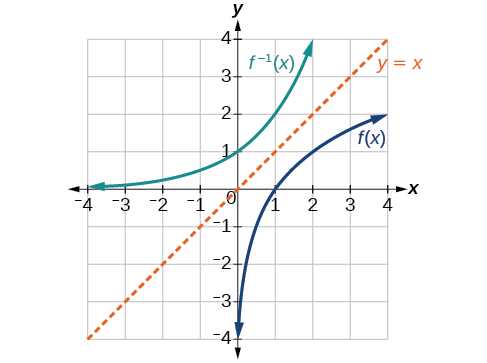# 3.7 Inverse functions  (Page 5/9)

 Page 5 / 9

Using the graph in [link] , (a) find $\text{\hspace{0.17em}}{g}^{-1}\left(1\right),$ and (b) estimate $\text{\hspace{0.17em}}{g}^{-1}\left(4\right).$

a. 3; b. 5.6

## Finding inverses of functions represented by formulas

Sometimes we will need to know an inverse function for all elements of its domain, not just a few. If the original function is given as a formula—for example, $\text{\hspace{0.17em}}y\text{\hspace{0.17em}}$ as a function of $\text{\hspace{0.17em}}x\text{—}$ we can often find the inverse function by solving to obtain $\text{\hspace{0.17em}}x\text{\hspace{0.17em}}$ as a function of $\text{\hspace{0.17em}}y.$

Given a function represented by a formula, find the inverse.

1. Make sure $\text{\hspace{0.17em}}f\text{\hspace{0.17em}}$ is a one-to-one function.
2. Solve for $\text{\hspace{0.17em}}x.$
3. Interchange $\text{\hspace{0.17em}}x\text{\hspace{0.17em}}$ and $\text{\hspace{0.17em}}y.$

## Inverting the fahrenheit-to-celsius function

Find a formula for the inverse function that gives Fahrenheit temperature as a function of Celsius temperature.

$C=\frac{5}{9}\left(F-32\right)$
$\begin{array}{ccc}\hfill C& =& \frac{5}{9}\left(F-32\right)\hfill \\ \hfill C\cdot \frac{9}{5}& =& F-32\hfill \\ \hfill F& =& \frac{9}{5}C+32\hfill \end{array}$

By solving in general, we have uncovered the inverse function. If

$C=h\left(F\right)=\frac{5}{9}\left(F-32\right),$

then

$F={h}^{-1}\left(C\right)=\frac{9}{5}C+32$

In this case, we introduced a function $\text{\hspace{0.17em}}h\text{\hspace{0.17em}}$ to represent the conversion because the input and output variables are descriptive, and writing $\text{\hspace{0.17em}}{C}^{-1}\text{\hspace{0.17em}}$ could get confusing.

Solve for $\text{\hspace{0.17em}}x\text{\hspace{0.17em}}$ in terms of $\text{\hspace{0.17em}}y\text{\hspace{0.17em}}$ given $\text{\hspace{0.17em}}y=\frac{1}{3}\left(x-5\right).$

$x=3y+5$

## Solving to find an inverse function

Find the inverse of the function $\text{\hspace{0.17em}}f\left(x\right)=\frac{2}{x-3}+4.$

So $\text{\hspace{0.17em}}{f}^{-1}\left(y\right)=\frac{2}{y-4}+3\text{\hspace{0.17em}}$ or $\text{\hspace{0.17em}}{f}^{-1}\left(x\right)=\frac{2}{x-4}+3.$

## Solving to find an inverse with radicals

Find the inverse of the function $\text{\hspace{0.17em}}f\left(x\right)=2+\sqrt{x-4}.$

$\begin{array}{ccc}\hfill y& =& 2+\sqrt{x-4}\hfill \\ \hfill {\left(y-2\right)}^{2}& =& x-4\hfill \\ \hfill x& =& {\left(y-2\right)}^{2}+4\hfill \end{array}$

So $\text{\hspace{0.17em}}{f}^{-1}\left(x\right)={\left(x-2\right)}^{2}+4.$

The domain of $\text{\hspace{0.17em}}f\text{\hspace{0.17em}}$ is $\text{\hspace{0.17em}}\left[4,\infty \right).\text{\hspace{0.17em}}$ Notice that the range of $\text{\hspace{0.17em}}f\text{\hspace{0.17em}}$ is $\text{\hspace{0.17em}}\left[2,\infty \right),\text{\hspace{0.17em}}$ so this means that the domain of the inverse function $\text{\hspace{0.17em}}{f}^{-1}\text{\hspace{0.17em}}$ is also $\text{\hspace{0.17em}}\left[2,\infty \right).$

What is the inverse of the function $\text{\hspace{0.17em}}f\left(x\right)=2-\sqrt{x}?$ State the domains of both the function and the inverse function.

${f}^{-1}\left(x\right)={\left(2-x\right)}^{2};\text{\hspace{0.17em}}\text{\hspace{0.17em}}\text{domain}\text{\hspace{0.17em}}\text{\hspace{0.17em}}\text{of}\text{\hspace{0.17em}}\text{\hspace{0.17em}}f:\text{\hspace{0.17em}}\text{\hspace{0.17em}}\left[0,\infty \right);\text{\hspace{0.17em}}\text{\hspace{0.17em}}\text{domain}\text{\hspace{0.17em}}\text{\hspace{0.17em}}\text{of}\text{\hspace{0.17em}}\text{\hspace{0.17em}}{f}^{-1}:\text{\hspace{0.17em}}\text{\hspace{0.17em}}\left(-\infty ,2\right]$

## Finding inverse functions and their graphs

Now that we can find the inverse of a function, we will explore the graphs of functions and their inverses. Let us return to the quadratic function $\text{\hspace{0.17em}}f\left(x\right)={x}^{2}\text{\hspace{0.17em}}$ restricted to the domain $\text{\hspace{0.17em}}\left[0,\infty \right)\text{,}$ on which this function is one-to-one, and graph it as in [link] .

Restricting the domain to $\text{\hspace{0.17em}}\left[0,\infty \right)\text{\hspace{0.17em}}$ makes the function one-to-one (it will obviously pass the horizontal line test), so it has an inverse on this restricted domain.

We already know that the inverse of the toolkit quadratic function is the square root function, that is, ${f}^{-1}\left(x\right)=\sqrt{x}.$ What happens if we graph both and ${f}^{-1}$ on the same set of axes, using the $x\text{-}$ axis for the input to both

We notice a distinct relationship: The graph of $\text{\hspace{0.17em}}{f}^{-1}\left(x\right)\text{\hspace{0.17em}}$ is the graph of $\text{\hspace{0.17em}}f\left(x\right)\text{\hspace{0.17em}}$ reflected about the diagonal line $\text{\hspace{0.17em}}y=x,\text{\hspace{0.17em}}$ which we will call the identity line, shown in [link] .Square and square-root functions on the non-negative domain

This relationship will be observed for all one-to-one functions, because it is a result of the function and its inverse swapping inputs and outputs. This is equivalent to interchanging the roles of the vertical and horizontal axes.

## Finding the inverse of a function using reflection about the identity line

Given the graph of $\text{\hspace{0.17em}}f\left(x\right)\text{\hspace{0.17em}}$ in [link] , sketch a graph of $\text{\hspace{0.17em}}{f}^{-1}\left(x\right).$

This is a one-to-one function, so we will be able to sketch an inverse. Note that the graph shown has an apparent domain of $\text{\hspace{0.17em}}\left(0,\infty \right)\text{\hspace{0.17em}}$ and range of $\text{\hspace{0.17em}}\left(-\infty ,\infty \right),\text{\hspace{0.17em}}$ so the inverse will have a domain of $\text{\hspace{0.17em}}\left(-\infty ,\infty \right)\text{\hspace{0.17em}}$ and range of $\text{\hspace{0.17em}}\left(0,\infty \right).$

If we reflect this graph over the line $\text{\hspace{0.17em}}y=x,\text{\hspace{0.17em}}$ the point $\text{\hspace{0.17em}}\left(1,0\right)\text{\hspace{0.17em}}$ reflects to $\text{\hspace{0.17em}}\left(0,1\right)\text{\hspace{0.17em}}$ and the point $\text{\hspace{0.17em}}\left(4,2\right)\text{\hspace{0.17em}}$ reflects to $\text{\hspace{0.17em}}\left(2,4\right).\text{\hspace{0.17em}}$ Sketching the inverse on the same axes as the original graph gives [link] .The function and its inverse, showing reflection about the identity line

#### Questions & Answers

write down the polynomial function with root 1/3,2,-3 with solution
if A and B are subspaces of V prove that (A+B)/B=A/(A-B)
write down the value of each of the following in surd form a)cos(-65°) b)sin(-180°)c)tan(225°)d)tan(135°)
Prove that (sinA/1-cosA - 1-cosA/sinA) (cosA/1-sinA - 1-sinA/cosA) = 4
what is the answer to dividing negative index
In a triangle ABC prove that. (b+c)cosA+(c+a)cosB+(a+b)cisC=a+b+c.
give me the waec 2019 questions
the polar co-ordinate of the point (-1, -1)
prove the identites sin x ( 1+ tan x )+ cos x ( 1+ cot x )= sec x + cosec x
tanh`(x-iy) =A+iB, find A and B
B=Ai-itan(hx-hiy)
Rukmini
what is the addition of 101011 with 101010
If those numbers are binary, it's 1010101. If they are base 10, it's 202021.
Jack
extra power 4 minus 5 x cube + 7 x square minus 5 x + 1 equal to zero
the gradient function of a curve is 2x+4 and the curve passes through point (1,4) find the equation of the curve
1+cos²A/cos²A=2cosec²A-1
test for convergence the series 1+x/2+2!/9x3ByByByBy